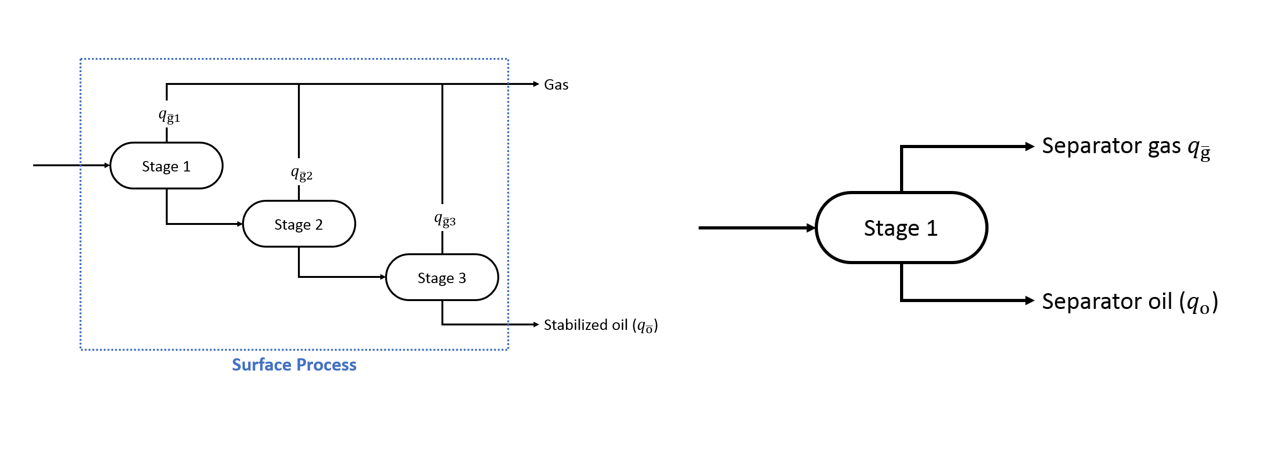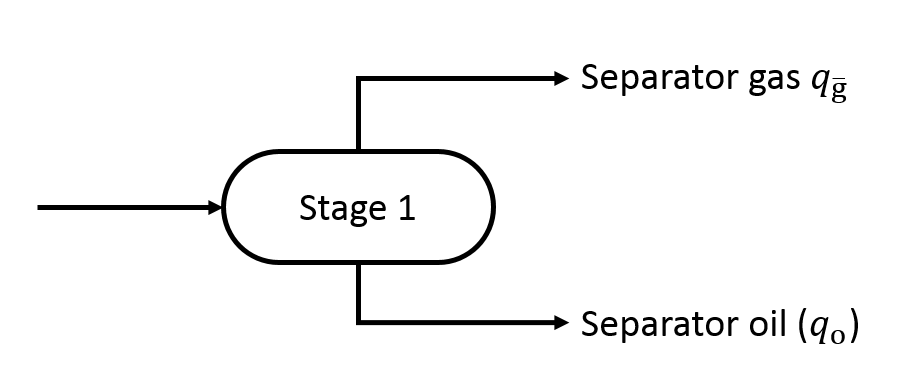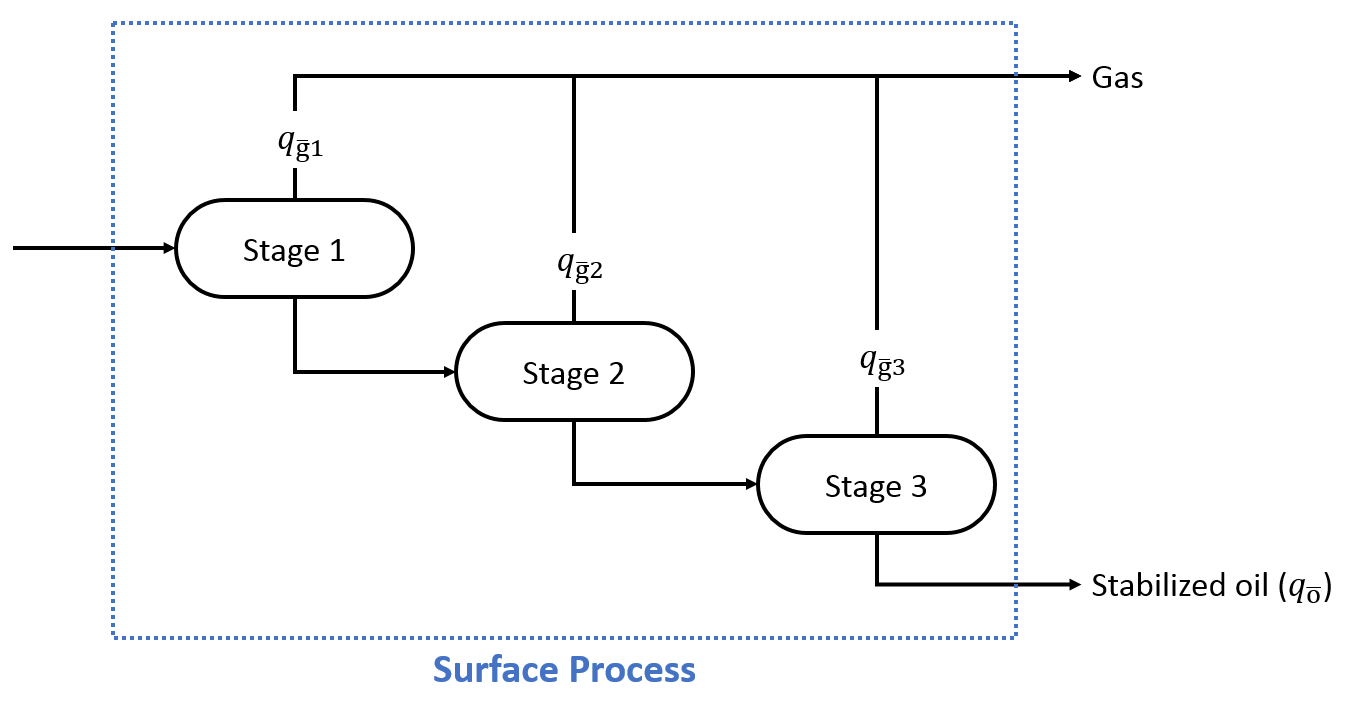# Fluid Definition Module

In this module, the user will input the fluid definition for a given well. The fluid definition is used consistenly across different modules of the software, where for a given well the fluid is the same for all calculations.

There are six methods for defining the insitu fluid of a well in whitson+:

Available Data Method Description
Initial GOR whitson+ calculates the initial composition based on initial GOR, the EOS model for the field / basin and a set of samples (or seed feeds) from the same field / basin.
Initial GOR and stock tank API whitson+ calculates the initial composition based on initial GOR, API, the EOS model for the field / basin and a set of samples (or seed feeds) from the same field / basin.
Initial GOR and saturation pressure whitson+ calculates the initial composition based on initial GOR, PSAT, the EOS model for the field / basin and a set of samples (or seed feeds) from the same field / basin.
Dry/Wet gas composition whitson+ uses the input composition up to $$C_{6+}$$ and split the $$C_{6+}$$ pseudo fraction using an exponential model using the input $$C_{6+}$$ MW to get the composition in the component slate of the EOS model
Separator oil and gas compositions whitson+ calculates the initial composition using the known (measured) separator oil and separator gas compositions, their corresponding initial producing separator GOR, the EOS model for the field / basin and a set of samples (or seed feeds) from the same field / basin.
Reservoir fluid composition whitson+ uses the specified composition as the composition of the well.

Which method to choose for a given well?

We recommend to always make use of as much of the available data as possible, as long as the data is quality checked and deemed appropriate for use in the calculations. The least data needed for a given well is the GOR, but for accurate predictions of the fluid we recommend also providing a stock tank oil API, which is easily obtained through field sampling.

The more data that becomes available, the more accurate the calculated initial reservoir composition should become. For further details about the data and the accuracy of the methods, see the methodology for wellstream composition estimation.

## Initial GOR

whitson+ calculates the initial composition based on initial GOR, the EOS model for the field / basin and a set of samples from the same field / basin. The GOR can be a total GOR or a GOR at separator conditions.

## Required Input Data

The data required to define the fluid in this case is:

• Initial GOR
• Reservoir temperature
• Initial reservoir pressure
• Surface process associated to the well

Note

The GOR can be a separator GOR or a full process GOR.In the case of a process GOR (left figure), the GOR is calculated as follows: In the case of a separator GOR (right figure), the GOR is given by: Note that the separator GOR is given in standard gas volume per separator oil volume (e.g. MMscf/sep. bbl), while the surface process GOR is given in standard volume per standard volume (e.g. MMscf/STB). Also Note that in whitson+ the last stage $$(p,T)$$ conditions are always the standard conditions (14.7 psia and 60 F).

### Estimation of the Initial Reservoir Fluid

The Regression on Fg method is used to estimate the in-situ reservoir composition $$z_{i}$$.

## Initial GOR and stock tank API

whitson+ calculates the initial composition based on initial GOR, API, the EOS model for the field / basin and a set of samples from the same field / basin.

### Required Input Data

The data required to define the fluid in this case is:

• Initial GOR (total process GOR or GOR at first-stage separataor)
• Initial stock tank oil API
• Reservoir temperature
• Initial reservoir pressure
• Surface process associated to the well
• (optional) Amounts of non-hydrocarbons
Why Isn't Gas Specific Gravity a Required Input?

Many conventional PVT correlations (e.g. correlations given by Standing) require gas specific gravity as an input. Hence, some people might wonder why gas specific gravity is not needed as an input here. The short answer is that when developing this method, we didn't find systematically better results when incorporating gas specific gravity as an input. This is because fluid type is not that correlated to gas specific gravity as for instance GOR and API, as seen in Table 2.1 in the SPE Phase Behavior.

Additionally, there is no need to have this additional datapoint, as it is already "implicitly" provided/constrained by the already available input data. How? Separator gas specific gravity can be calculated from the separator gas compositions. Separator gas compositions is found by multiplying the equilibrium ratios (K-values = yi/xi) provided by the EOS model with the separator oil composition. The separator oil composition is estimated from the the liquid API and separator conditions.

Note

If the amounts of non-hydrocarbons are not specified by the user, the calculated composition will inherit these amounts from the selected seed feed composition, which are generally average values in the given field or basin. We recommend specifying these amounts if the variation of non-hydrocarbons amounts is large in your field, for accurate description of these components in the calculated composition.

### Estimation of the Initial Reservoir Fluid

The API Interpolation / Fg regression is used to estimate the in-situ reservoir composition $$z_{i}$$.

## Initial GOR and Saturation Pressure

whitson+ calculates the initial composition based on initial GOR, PSAT, the EOS model for the field / basin and a set of samples from the same field / basin.

### Required Input Data

The data required to define the fluid in this case is:

• Initial GOR (total process GOR or GOR at first-stage separataor)
• Saturation pressure
• Reservoir temperature
• Initial reservoir pressure
• Surface process associated to the well
• (optional) Amounts of non-hydrocarbons

### Estimation of the Initial Reservoir Fluid

The PSAT Interpolation / Fg regression is used to estimate the in-situ reservoir composition $$z_{i}$$.

## Dry or Wet Gas Composition

whitson+ calculates the initial composition based on the specified dry/wet gas reservoir fluid composition up to $$C_{6+}$$ and the input $$C_{6+}$$ MW. The input composition is honored exactly and the $$C_{6+}$$ fraction total amount is split into heavier fractions (defined in the EOS model) using an exponential molar distribution model knowing the $$C_{6+}$$ MW.

## Required Input Data

The data required to define the fluid in this case is:

• Dry/wet gas composition up to $$C_{6+}$$
• Reservoir temperature
• Initial reservoir pressure
• $$C_{6+}$$ MW

## Separator Oil and Gas Compositions

whitson+ calculates the initial composition using the known (measured) separator oil and separator gas compositions, their corresponding initial producing separator GOR, the EOS model for the field / basin and a set of samples (or seed feeds) from the same field / basin.

### Required Input Data

The data required to define the fluid in this case includes:

• Initial separator (well test) GOR
• Measured composition of separator oil and separator gas (up to $$\mathrm{C_{7+}}$$)
• $$\mathrm{C_{7+}}$$ molecular weights (for both separator oil and separator gas)
• Separator $$(p, T)$$ conditions
• Reservoir temperature
• Initial reservoir pressure
• (Optional) Stock tank liquid API

An example dataset is provided here in PDF format and here in Excel format

Note

Note that separator GOR is the "well test GOR" measured right after the separator:The separator GOR is computed as follows: and is given in scf/sep. bbl (or sm³/sep. m³).
Note that the oil rate is at separator conditions, i.e. without applying a shrinkage factor.

When stock tank liquid API is important?

It is important to note that the stock tank liquid API referred here is the API measured on the stock tank oil from the single stage (atmospheric) flash of the collected separator oil in the PVT lab. The calculated composition without stock tank liquid API input should be accurate if the $$\mathrm{C_{7+}}$$ of separator oil is relaible from the PVT lab. If the lab reported separator oil $$\mathrm{C_{7+}}$$ is not reliable then it is recommended to specify the measured stock tank oil API. In that case, whitson+ will estimate the $$\mathrm{C_{7+}}$$ molecular weight honoring the input API. The estimated $$\mathrm{C_{7+}}$$ molecular weight is then used to extend the $$\mathrm{C_{7+}}$$ composition to EOS $$\mathrm{C_{N+}}$$ (e.g. $$\mathrm{C_{36+}}$$).

Why does whitson+ need separator oil $$\mathrm{C_{7+}}$$ molecular weight when user inputs stock tank liquid API?

Separator oil $$\mathrm{C_{7+}}$$ molecular weight is used in whitson+ for two different purposes; (i) converting the lab reported molar composition to lab measured mass composition and (ii) extending the $$\mathrm{C_{7+}}$$ composition to EOS $$\mathrm{C_{N+}}$$ (e.g. $$\mathrm{C_{36+}}$$). If the lab $$\mathrm{C_{7+}}$$ is not reliable, this would mean that the lab reported molar composition of separator oil up to $$\mathrm{C_{7+}}$$ is wrong due to wrong (unreliable) $$\mathrm{C_{7+}}$$ molecular weight being used to convert the reliable measured mass composition to reported molar composition. Therefore, lab $$\mathrm{C_{7+}}$$ molecular weight is always needed (and used) to convert back the lab reported molar composition to measured mass composition up to $$\mathrm{C_{7+}}$$. For (ii), the lab reported $$\mathrm{C_{7+}}$$ molecular weight is used if stock tank oil API is not input, otherwise the estimated $$\mathrm{C_{7+}}$$ molecular weight is used honoring the input API.

### Estimation of the Initial Reservoir Fluid

The comprehensive separator data matching method is used to estimate the in-situ reservoir composition $$z_{i}$$.

## Reservoir Fluid Composition

whitson+ uses the specified composition as the composition of the well.

### Required Input Data

The only data required to define the fluid in this case is the fluid composition. The composition should be provided in the same compositional slate as the EOS model associated to the field for the well in question.

### Estimation of the Initial Reservoir Fluid

There is no estimation of the initial reservoir fluid in this case, as the provided composition is already the initial reservoir fluid to be used in calculation.

## Define Surface Process

Each well in whitson+ has a surface process associated to it. This surface process is used in different modules, wherever a surface process is required (e.g. black oil tables or defining the insitu fluid from API and GOR).Multiple wells can share the same surface process, and the connection is done through the name of the process. This means that when two wells have the same process name, they are using the same process and updating the process for one well will affect the other well too.

It is at any given time possible to link and de-link wells to a surface process, as well as change process conditions or number of stages.

## Phase Envelope Generation

Given a feed composition ($$z_i$$) and equation of state (EOS) fluid model, a pressure-temperature phase envelope is generated yielding the pressure-temperature boundary of the two-phase region and the associated fluid type of the boundary (bubble-point or dew-point). In addition to the phase boundary, the phase envelope estimates the critical point (if one exists). To give a reference to the initial reservoir fluid classification, the initial reservoir conditions are also plotted with the phase envelope.

## Export Formats

The phase envelope can be exported as either (1) only a figure or (2) a figure with the associated data. The "export to figure" option gives the option of either a PNG or SVG file. PNG files are a standard figure format, while a SVG files are so-called vector-format files (e.g. enhanced metafile in Microsoft Office products).

Note

If you are planing on using PowerPoint (or other Microsoft Office products), we suggest using the SVG file. The reason for this is that it gives better resolution (because of the vector format).

The "export to Excel" option automatically generates an Excel file with the data in the phase envelope figure and a separate worksheet with the figure.